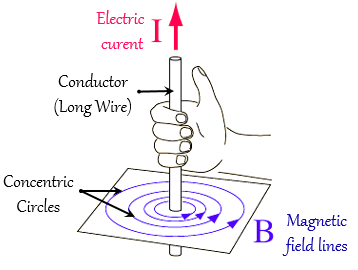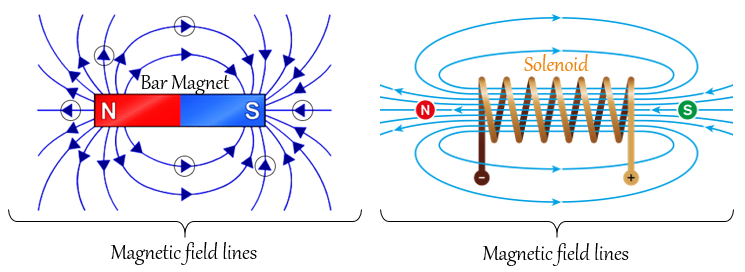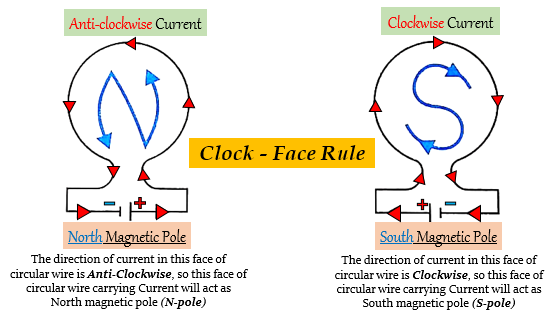# Fill in the following blanks with suitable words:(a) The lines of _______ round a straight current-carrying conductor are in the shape of _______.(b) For a current-carrying solenoid, the magnetic field is like that of a _______.(c) The magnetic effect of a coil can be increased by increasing the number of _______, increasing the _______, or inserting an _______ core.(d) If a coil is viewed from one end and the current flows in an anticlockwise direction, then this end is a _______ pole.(e) If a coil is viewed from one end, and the current flows in a clockwise direction, then this end is a _______ pole.

(a) The lines of magnetic field round a straight current-carrying conductor are in the shape of concentric circles.

Explanation(b) For a current-carrying solenoid, the magnetic field is like that of a bar magnet.

Explanation
The magnetic field produced by a current-carrying solenoid resembles the magnetic field pattern produced by a bar magnet.(c) The magnetic effect of a coil can be increased by increasing the number of turns increasing the current or inserting an iron core.

Explanation
The three ways in which the strength of an electromagnet can be increased are:
1. By increasing the number of turns in the coil of the electromagnet.
2. By increasing the current flowing through the coil of the electromagnet.
3. By reducing the length of the air gap present between the poles of the electromagnet .

(d) If a coil is viewed from one end and the current flows in an anticlockwise direction, then this end is a north pole.

Explanation
The polarity of the coil will be given by the clock rule. When we face the one end of the coil and if the current flows anti-clockwise, then the end of the coil will act as the North Pole and the other end will be the South Pole.

(e) If a coil is viewed from one end, and the current flows in a clockwise direction, then this end is a south pole.

Explanation
The polarity of the coil will be given by the clock face rule. When we face the one end of the coil and if the current flows clockwise, then the end of the coil will act as the South Pole and the other end will be the North Pole.

According to this rule, if we look at one face of the circular wire (or coil) through which a current is passing, we have the following conclusions:
1. If the current around the front face of the circular wire (or coil) flows in the Clockwise direction, then this face of the circular wire will be the South pole (S-pole).
And, the flow of current in the back face of the circular wire will be Anticlockwise and acts as a North pole.

2. If the current around the front face of the circular wire (or coil) flows in the Anticlockwise direction, then this face of the circular wire will be the North pole (N-pole).
And, the flow of current in the back face of the circular wire will be Clockwise and acts as a South pole.Updated on: 10-Oct-2022

91 Views